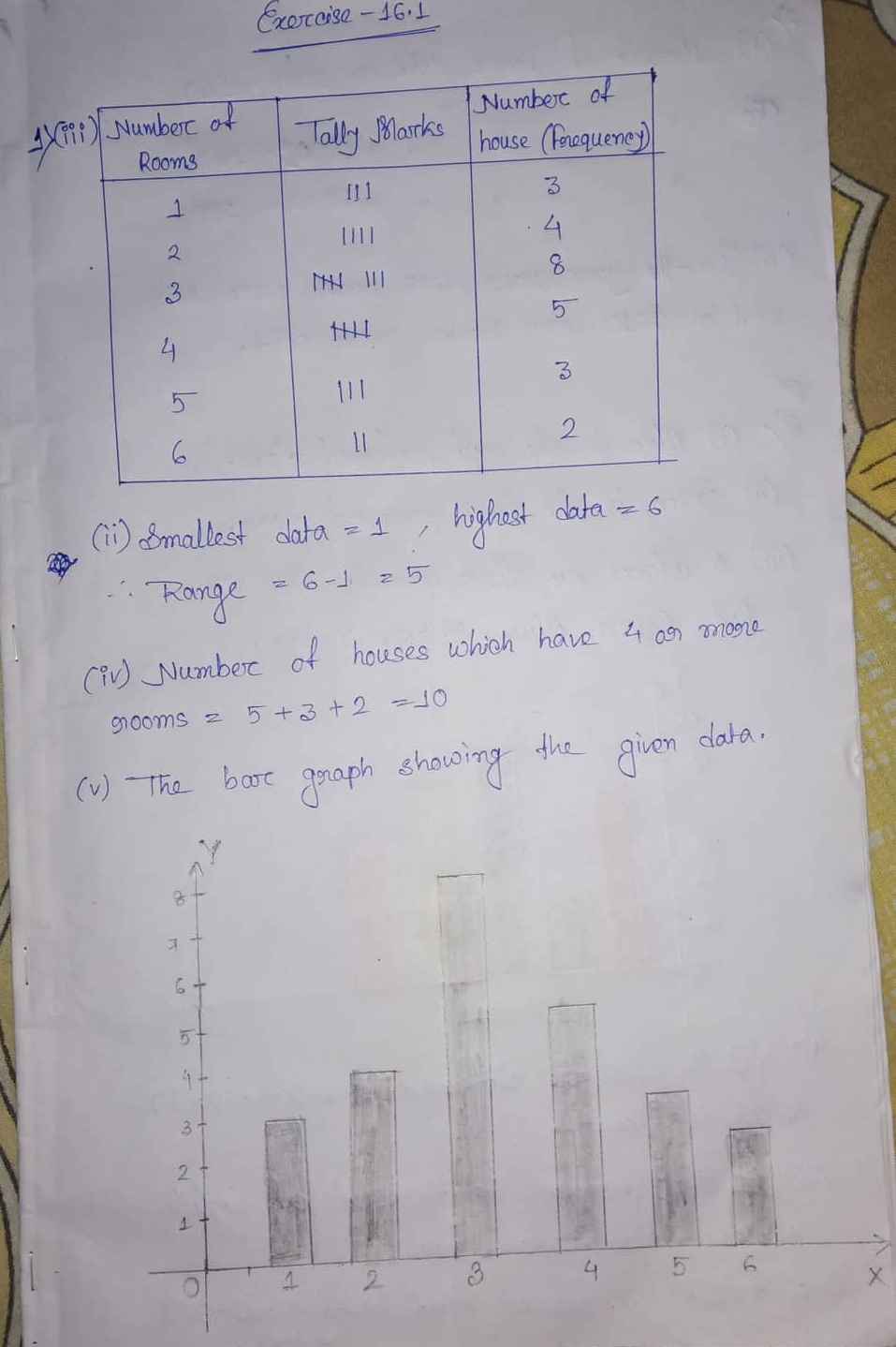# ML Aggarwal CBSE Solutions Class 7 Math Sixteenth Chapter Data Handling Exercise 16.1

### ML Aggarwal CBSE Solutions Class 7 Math 13th Chapter Data Handling Exercise 16.1

(1) The number of rooms in 25 houses of a locality is as given below:

4,3,2,6,4,3,2,1,5,3,2,3,4,3,5,1,6,1,3,4,2,3,4,3,5

(i) Arrange the above data in ascending order.

(ii) Find the range of the data.

(iii) Construct frequency distribution table for the above data.

(iv) Find the number of houses which have 4 or more than 4 rooms.

(v) Draw bar graph to represent the above data.

Solution:(2) The adjoining bar graph shows the number of books sold by a bookstore during five consecutive years. Read the bar graph and answer the following questions:

(i) About how many books were sold in 2008, 2009 and 2011 years?

(ii) In which years about 475 books and 225 books sold?

(3) Two hundred students of 6th and 7th class were asked to name their favorite colour so as to decide upon what should be the colour of their school buiding . The results are shown in the following table:

 Favorite colour red green blue yellow orange Number of students 43 19 55 49 34

Represents the given data on a bar graph.

Answer the following questions with the help of bar graph:

(i) Which is the most preferred colour?

(ii) Which is the least preferred colour?

(iii) How many colours are there in all? What are they?

(4) (4) Sale of English and Hindi books in four consecutive years is given below:

 Years 2008 2009 2010 2011 English 350 400 450 620 Hindi 500 525 600 650

Draw a double bar graph to represent the above data and answer the following questions:

(i) In which year was the difference in the sale of two language books least?

(ii) Can you say that the demand for English books rose faster? Justify your answer.

(5) Consider the following data collected from a survey of a colony:

 Favorite sport cricket basketball swimming hocky atheletics Watching 1240 470 510 430 250 participating 620 320 320 250 110

Draw a double bar graph choosing an appropriate scale.

Answer the following questions using the bar graph:

(i) Which sports is most popular?

(ii) Which is more preferred, watching or participating in sports?

Solution:Updated: November 27, 2019 — 2:29 am